top of page
Search

# National 5 Maths Formula Sheet with notes and examples

Updated: Apr 6, 2022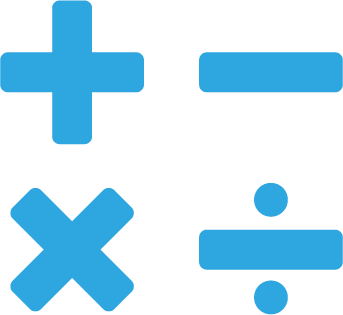There's the National 5 Maths Formula Sheet that you'll find in the SQA exam, and on some websites, and then there's our National 5 Maths Formula Sheet! Ours has been specially made to provide extra support to National 5 Maths students to explain which formulae you need to know and remember and which key areas from the course it is linked to. We've even provided examples from past paper questions where it applies, and of course, the solutions!This formula is used when you are asked to solve a quadratic equation, the clue that you should use this formula; is that you are asked to round your answers, usually to 1 or 2 decimal places. Even if you can solve the quadratic equation by factorising, you can still use this formula to solve, it will give your final values of x.

Occasionally, this formula is used in the non-calculator paper. If so, you may need to leave your answers as surds.

Past paper example with the quadratic formula:Triangle Calcualtions - The Sine RuleThis formula is used to calculate the length of sides and the sizes of angles in a triangle.

To use this formula, you need to know 1 pair of a side and opposite angle and one other side or angle.

When using this formula to calculate an angle you can turn it upside down.

This makes the re-arranging step easier:(This is just the sine rule upside down)

Past paper example with the sine rule:Another past paper example with the sine rule:The Cosine Rule – for a sideThis formula is used to calculate the length of sides of a triangle. You can use this formula to find the length of a 3rd side of a triangle if you know the other two sides and the angle in-between them.

Past paper example with the cosine rule:The Cosine Rule – for an angleThis formula is used to calculate the size of angles in a triangle.

You can use this formula to find the size of an angle when you know all 3 sides of a triangle.

Past paper example with the cosine rule: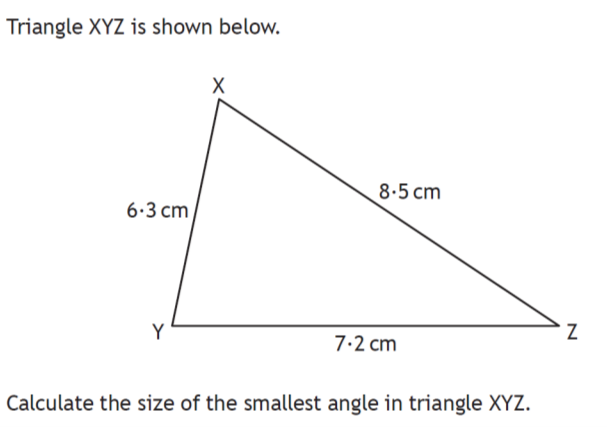The Area of a TriangleThis formula is used to calculate the area of a triangle.

This can often be in a non-calculator paper and often within problem solving questions.

Past paper example with area of a triangle:Another past paper example with area of a triangle: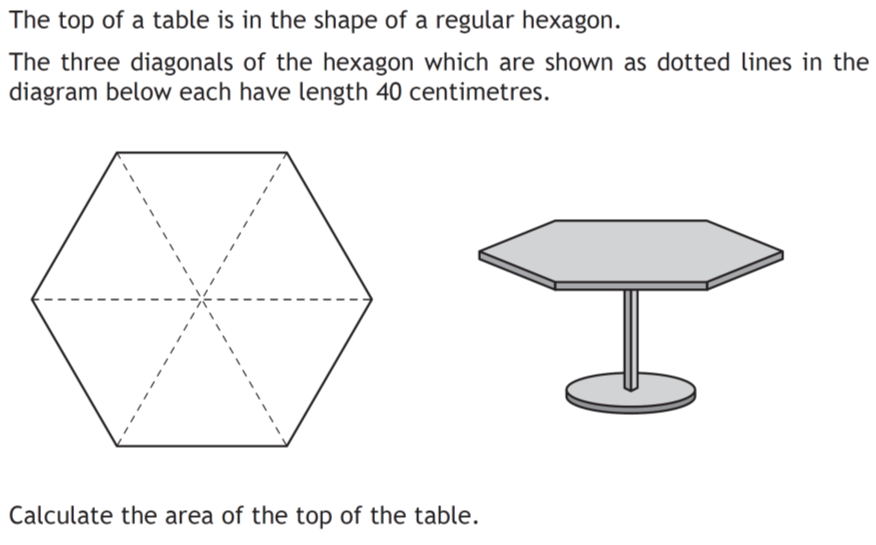Volume calculations

Volume of a sphereThis formula is used to calculate the volume of a sphere.

It is very common to have to find the volume of a hemisphere (half a sphere).

You can either use:orVolume of a coneThis formula is used to calculate the volume of a cone.

This formula is useful to remember the volume of a cylinder which is not given.

To find the volume of a cylinder, just remove the third to give: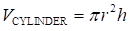Volume of a pyramidThis formula is used to calculate the volume of a pyramid and does not come up very often.

Many volume questions are composite volume questions. This means two different volumes added or subtracted. Most volume questions include a significant figure rounding. Always make sure that you include the units in your final answer.

Past paper example:In volume calculations you can be asked to use the volume formulae to calculate other dimensions of 3D shapes.

Past paper example (non-calculator):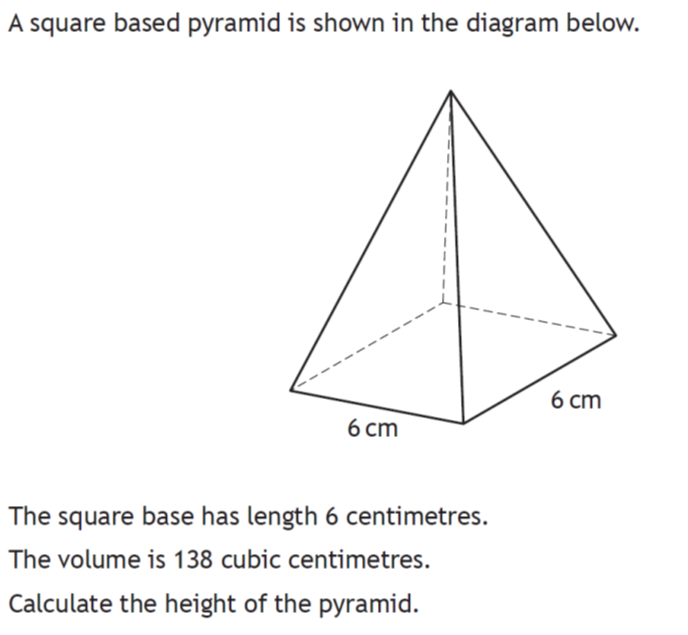orStandard deviationA very common question is to find the mean and the standard deviation.

Depending on how you were taught you would use one of these two formulae.

Past paper example on standard deviation: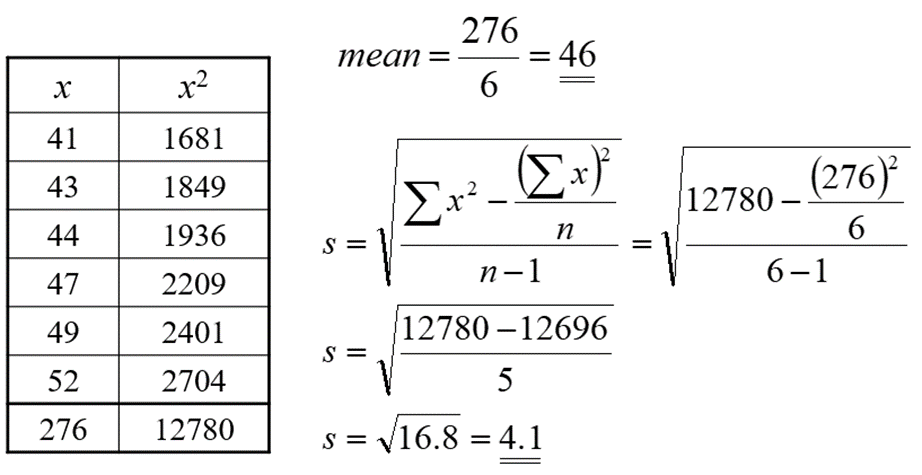Another past paper example on standard deviation:If you need support in National 5 or Higher subjects you can book classes here.